MatLab Toolbox - LTE                                      Home : www.sharetechnote.com

PHICH

If you don't know what PHICH(Physical Hybrid ARQ Indicator Channel) is, refer to Physical Layer Channel : Downlink : PHICH(Physical Hybrid ARQ Indicator Channel) page first.

< PHICH Parameter Calculation >

The PHICH related paremeter configured by RRC message (MIB in this case) is only two as shown below in MIB,but many other parameter are derived from that single infomration element in MIB and other indirectly related parameter (like System BW). so it would be helpful to see what kind of intermediate parameters are deribed before we generate physical symbols. This section shows the list of intermediate parameters and their values from eNodeB parameters.

% First you have to define properites of a eNodeB.

% NDLRB indicate System Bandwith in the unit of RBs.

% NDLRB 6 = 1.4 Mhz, NDLRB 15 = 3.0 Mhz, NDLRB 25 = 5.0 Mhz,

% NDLRB 50 = 10 Mhz, NDLRB 75 = 15 Mhz, NDLRB 100 = 20 Mhz

% CellRefP indicate number of downlink Antenna. CellRefP = 1 means 1 transmission antenna (SISO)

% NCellID indicate PCI (Physical Channel Identity) of the Cell

% NSubframe indicate the subframe number.

enb.CyclicPrefix = 'Normal';

enb.PHICHDuration = 'Normal';

enb.Ng = 'One';

enb.NDLRB = 6;

enb.CellRefP = 1;

enb.DuplexMode = 'FDD';

enb.NCellID = 0;

enb.NSubframe = 0;

enb.CFI = 1;

% Once you configured eNB, just pass it to ltePHICHInfo() function and it will give you all the intermediate parameters that are required to generate PHICH symbol and allocate them onto resource grid.

phichInfo = ltePHICHInfo(enb);

Following four examples shows the result by changing only Ng value while keeping every other parameters same. See what has changed in result and see if you can explain to yourself on why you have this kind of result.

 enb.NDLRB = 6 enb.CellRefP = 1 enb.Ng = 'Sixth' enb.NDLRB = 6 enb.CellRefP = 1 enb.Ng = 'Half' enb.NDLRB = 6 enb.CellRefP = 1 enb.Ng = 'One' enb.NDLRB = 6 enb.CellRefP = 1 enb.Ng = 'Two' NREG:               NRE:            NPHICH:           NGroups:     NMappingUnits:        NSequences:     PHICHDuration: 3 12 8 1 1 8 1 3 12 8 1 1 8 1 3 12 8 1 1 8 1 6 24 16 2 2 8 1

Following four examples shows the result by changing only NDLRB value while keeping every other parameters same. See what has changed in result and see if you can explain to yourself on why you have this kind of result.

 enb.NDLRB = 6 enb.CellRefP = 1 enb.Ng = 'Sixth' enb.NDLRB = 25 enb.CellRefP = 1 enb.Ng = 'Sixth' enb.NDLRB = 50 enb.CellRefP = 1 enb.Ng = 'Sixth' enb.NDLRB = 100 enb.CellRefP = 1 enb.Ng = 'Sixth' NREG:               NRE:            NPHICH:           NGroups:     NMappingUnits:        NSequences:     PHICHDuration: 3 12 8 1 1 8 1 3 12 8 1 1 8 1 6 24 16 2 2 8 1 9 36 24 3 3 8 1

Following four examples shows the result by changing only CellRefP  value while keeping every other parameters same. See what has changed in result and see if you can explain to yourself on why you have this kind of result.

 enb.NDLRB = 6 enb.CellRefP = 1 enb.Ng = 'Sixth' enb.NDLRB = 6 enb.CellRefP = 2 enb.Ng = 'Sixth' enb.NDLRB = 6 enb.CellRefP = 3 enb.Ng = 'Sixth' enb.NDLRB = 6 enb.CellRefP = 4 enb.Ng = 'Sixth' NREG:               NRE:            NPHICH:           NGroups:     NMappingUnits:        NSequences:     PHICHDuration: 3 12 8 1 1 8 1 3 12 8 1 1 8 1 3 12 8 1 1 8 1 6 24 16 2 2 8 1

< PHICH Symbol >

This section shows you how you can generate physical symbols for PHICH channel.

% First you have to define properites of a eNodeB.

% NDLRB indicate System Bandwith in the unit of RBs.

% NDLRB 6 = 1.4 Mhz, NDLRB 15 = 3.0 Mhz, NDLRB 25 = 5.0 Mhz,

% NDLRB 50 = 10 Mhz, NDLRB 75 = 15 Mhz, NDLRB 100 = 20 Mhz

% CellRefP indicate number of downlink Antenna. CellRefP = 1 means 1 transmission antenna (SISO)

% NCellID indicate PCI (Physical Channel Identity) of the Cell

% NSubframe indicate the subframe number.

enb.CyclicPrefix = 'Normal';

enb.PHICHDuration = 'Normal';

enb.Ng = 'Sixth';

enb.NDLRB = 6;

enb.CellRefP = 4; % This is the case for 4 Antenna Port. You can set it to be '1' for Single Antenna Case

enb.DuplexMode = 'FDD';

enb.NCellID = 0;

enb.NSubframe = 0;

enb.CFI = 1;

% Since the purpose of PHICH is to transmit 'ACK' or 'NACK' information, you have to configure whether

% you want to send ACK or NACK. Also you have many choce to carry this ACK/NACK information in terms of

% PHICH Group Index and Sequence Number. So you have to configure these values before you generate

% a specific PHICH Symbol sequence.

PHICH_Group_Index = 0;

PHICH_Sequence_Index = 1;

HARQ_Indicator_Value = 0; % 0 = NACK, 1 = ACK

% Once you have configured all the parameters for PHICH, just pass them to the fuction ltePHICH() and it will

% generate the physical symbols. phichSymbol_rows, phichSymbol_cols, phichSymbol_row_index are only for

% plotting (visualization) at later steps.

phichSymbol = ltePHICH(enb,[PHICH_Group_Index,PHICH_Sequence_Index,HARQ_Indicator_Value]);

phichSymbol_rows= length(phichSymbol(:,1));

phichSymbol_cols = length(phichSymbol(1,:));

phichSymbol_row_index = 0:phichSymbol_rows-1;

% Following is to plot the PHICH symbol transmitted by the first antenna port.

subplot(phichSymbol_cols,3,1);

plot(real(phichSymbol(:,1)),imag(phichSymbol(:,1)),'ro','MarkerFaceColor',[1 0 0]);

title('Constellation');

subplot(phichSymbol_cols,3,[2 3]);

plot(phichSymbol_row_index,real(phichSymbol(:,1)),'ro-',phichSymbol_row_index,imag(phichSymbol(:,1)),'bo-');

xlim([0 max(phichSymbol_row_index)]);

title('PHICH index vs PHICH value. Red -> real, Blue -> Imaginary');

% Following is to plot the PHICH symbol transmitted by the second antenna port if it is used.

if enb.CellRefP >= 2

subplot(phichSymbol_cols,3,4);

plot(real(phichSymbol(:,2)),imag(phichSymbol(:,2)),'ro','MarkerFaceColor',[1 0 0]);

title('Constellation');

subplot(phichSymbol_cols,3,[5 6]);

plot(phichSymbol_row_index,real(phichSymbol(:,2)),'ro-',phichSymbol_row_index,imag(phichSymbol(:,2)),'bo-');

xlim([0 max(phichSymbol_row_index)]);

title('PHICH index vs PHICH value. Red -> real, Blue -> Imaginary');

end

% Following is to plot the PHICH symbol transmitted by the third antenna port if it is used

if enb.CellRefP >= 3

subplot(phichSymbol_cols,3,7);

plot(real(phichSymbol(:,3)),imag(phichSymbol(:,3)),'ro','MarkerFaceColor',[1 0 0]);

title('Constellation');

subplot(phichSymbol_cols,3,[8 9]);

plot(phichSymbol_row_index,real(phichSymbol(:,3)),'ro-',phichSymbol_row_index,imag(phichSymbol(:,3)),'bo-');

xlim([0 max(phichSymbol_row_index)]);

title('PHICH index vs PHICH value. Red -> real, Blue -> Imaginary');

end

% Following is to plot the PHICH symbol transmitted by the fourth antenna port if it is used

if enb.CellRefP >= 4

subplot(phichSymbol_cols,3,10);

plot(real(phichSymbol(:,4)),imag(phichSymbol(:,4)),'ro','MarkerFaceColor',[1 0 0]);

title('Constellation');

subplot(phichSymbol_cols,3,[11 12]);

plot(phichSymbol_row_index,real(phichSymbol(:,4)),'ro-',phichSymbol_row_index,imag(phichSymbol(:,4)),'bo-');

xlim([0 max(phichSymbol_row_index)]);

title('PHICH index vs PHICH value. Red -> real, Blue -> Imaginary');

end

Following example shows you four different sequence of PHICH symbols (12 symbols always) on each of the four antenna.

 PHICH_Group_Index = 0; PHICH_Sequence_Index = 2; HARQ_Indicator_Value = 0; enb.CellRefP = 4 If you set enb.CellRefP = 1, you would have only one sequence of data. Ecah of the following sequence will be allocated to each of the four antenna port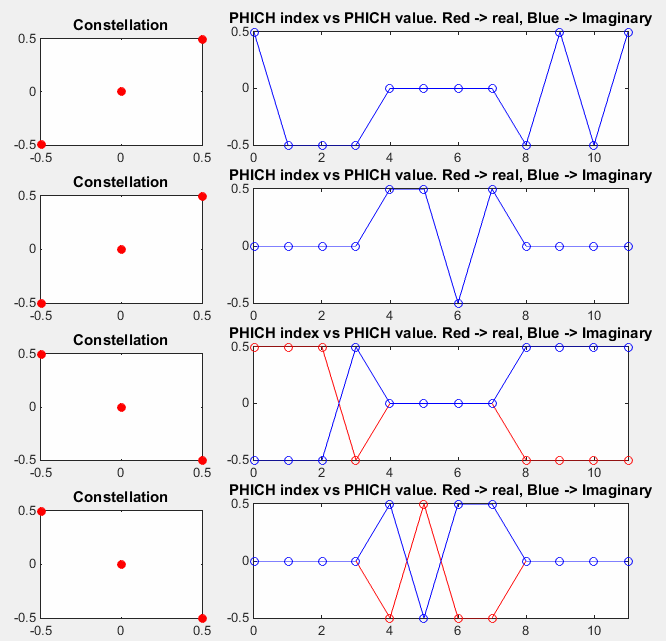Following example shows you four different sequence of PHICH symbols (12 symbols always) on each of the four antenna. The only difference from previous example is HARQ_Indicator_Value. Previous is for NACK and this example is for ACK.

 PHICH_Group_Index = 0; PHICH_Sequence_Index = 2; HARQ_Indicator_Value = 1; enb.CellRefP = 4 If you set enb.CellRefP = 1, you would have only one sequence of data. Ecah of the following sequence will be allocated to each of the four antenna port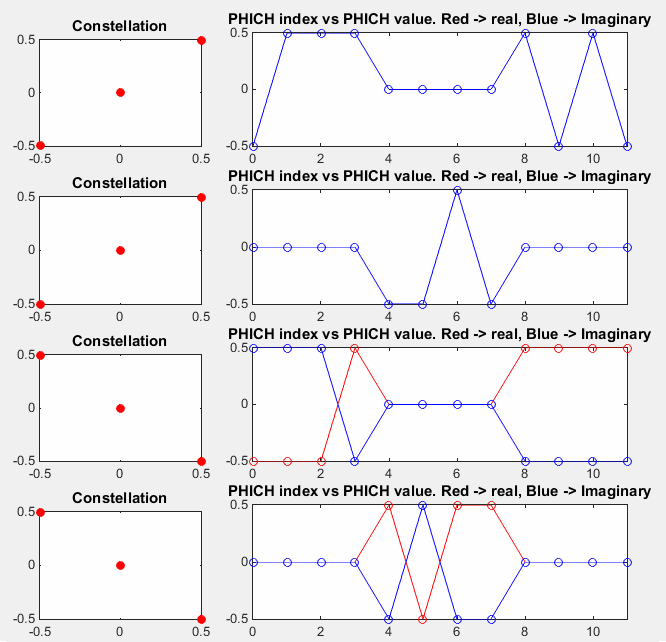This example is for PHICH for sending NACK which is similar to the first example. The only difference between this example and the first example is PHICH_Sequence_Index

 PHICH_Group_Index = 0; PHICH_Sequence_Index = 1; HARQ_Indicator_Value = 0; enb.CellRefP = 4 If you set enb.CellRefP = 1, you would have only one sequence of data. Ecah of the following sequence will be allocated to each of the four antenna port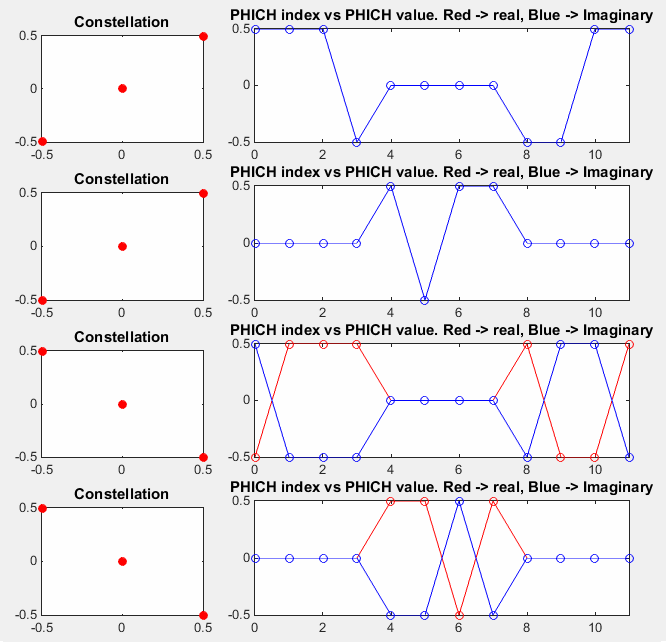< PHICH RE Mapping>

The last step is to allocate each of the symbols to corresponding Resource Elements and this section shows the matlab code for that process.

% First you have to define properites of a eNodeB.

% NDLRB indicate System Bandwith in the unit of RBs.

% NDLRB 6 = 1.4 Mhz, NDLRB 15 = 3.0 Mhz, NDLRB 25 = 5.0 Mhz,

% NDLRB 50 = 10 Mhz, NDLRB 75 = 15 Mhz, NDLRB 100 = 20 Mhz

% CellRefP indicate number of downlink Antenna. CellRefP = 1 means 1 transmission antenna (SISO)

% NCellID indicate PCI (Physical Channel Identity) of the Cell

% NSubframe indicate the subframe number.

enb.CyclicPrefix = 'Normal';

enb.PHICHDuration = 'Normal';

enb.Ng = 'Sixth';

enb.NDLRB = 6;

enb.CellRefP = 1;

enb.DuplexMode = 'FDD';

enb.NCellID = 0;

enb.NSubframe = 0;

enb.CFI = 1;

% Following is to create an empty resource grid for one subframe.

resourceGrid = lteDLResourceGrid(enb);

% Following is to create symbols for Cell Specific Reference Signal and make a list of resource index for the

% reference signal.

rs_Ant0_sym = lteCellRS(enb,0);

rs_Ant0_sym_ind = lteCellRSIndices(enb,0);

rs_Ant0_arrayIndex = 0:length(rs_Ant0_sym)-1;

% Following is to create symbols for PCFICH and make a list of resource index for the signal

cfi_cw = lteCFI(enb);

pcfich_sym = ltePCFICH(enb,cfi_cw);

pcfich_sym_arrayIndex = 0:length(pcfich_sym)-1;

pcfich_sym_ind = ltePCFICHIndices(enb,{'1based','re'});

phich_sym_ind = ltePHICHIndices(enb,{'1based','re'});

% Following is to create symbols for PSS and make a list of resource index for the signal

pss = ltePSS(enb);

pss_arrayIndex = 0:length(pss)-1;

pss_sym_ind = ltePSSIndices(enb,0,{'1based','re'});

% Following is to create symbols for SSS and make a list of resource index for the signal

sss = lteSSS(enb);

sss_arrayIndex = 0:length(sss)-1;

sss_sym_ind = lteSSSIndices(enb,0,{'1based','re'});

% Following part is filling the resource grid with each of the signal.. but if you see carefully I didn't fill this

% with real symbol number, I just filled it with a constant that I arbitrarily set. This is just for visualization..

% just to allocate constant/outstanding color for each signal. When you  use this resource grid for real

% transmission (not for visualization), fill the resourceGrid with real symbol value you generated above.

pss_scale = 0.2;

sss_scale = 0.4;

rs_scale = 1.0;

pcfich_scale = 0.5;

phich_scale = 0.8;

resourceGrid(pss_sym_ind) = pss_scale;

resourceGrid(sss_sym_ind) = sss_scale;

resourceGrid(rs_Ant0_sym_ind) = rs_scale;

resourceGrid(pcfich_sym_ind) = pcfich_scale;

resourceGrid(phich_sym_ind) = phich_scale;

% Following is to display the resource grid. I didn't find any proper functions in the toolbox to display

% one subframe grid as I like. So I used a little bit of tricks. First I plot 3D surface graph with the grid and

% move the view point right on top of the plot so that it looks like plane 2D grid.

xStep = 0:13;

yStep = 0:(enb.NDLRB*12-1);

surface(xStep,yStep,abs(resourceGrid));

axis([0 13 0 (enb.NDLRB*12-1) 0 1]);

view([0,90]);

set(gca,'xtick',[0 6 7 13]);

set(gca,'ytick',[[0:12:enb.NDLRB*12-1] [enb.NDLRB*12-1]]);

Followings are the two example of allocating PHICH for two difference cell configuration. The only difference between the two is NCellID (PCI). The orange color in the symbol 0 represents PHICH. As you see, the number of the orange RE is always 12, but the location changes depending NCellID.

 enb.NCellID = 0; enb.CellRefP = 1; enb.PHICHDuration = 'Normal'; enb.Ng = 'Sixth'; enb.NDLRB = 6; enb.CFI = 1; enb.NCellID = 1; enb.CellRefP = 1; enb.PHICHDuration = 'Normal'; enb.Ng = 'Sixth'; enb.NDLRB = 6; enb.CFI = 1;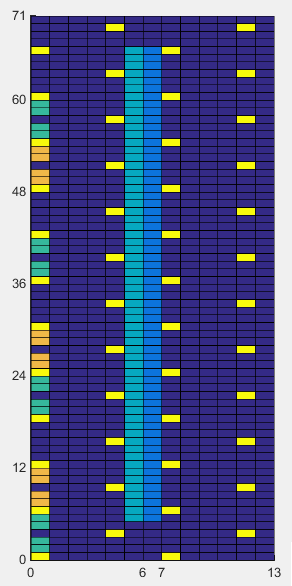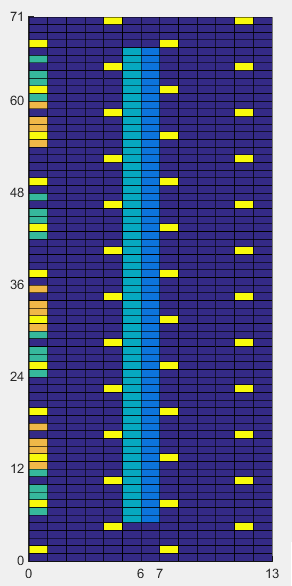Followings are two example of PHICH allocation for the same system bandwidth of 5 Mhz. The only difference between these two examples is Cell ID. The difference between this example and previous example is system bandwidth. The PHICH position is the 'orange' resource elements in the first symbol. If you count the number of green REs, it is same as in previous example. It means the number of PHICH RE does not change with system BW. But you would notice the gap between each PHICH REG(Resource Element Group) are different from previous example. Basic idea for PHICH resource allocation is to distribute the 3 REGs as evenly as possible accross full system bandwidth.

 enb.NCellID = 0; enb.CellRefP = 1; enb.PHICHDuration = 'Normal'; enb.Ng = 'Sixth'; enb.NDLRB = 25; enb.CFI = 1; enb.NCellID = 1; enb.CellRefP = 1; enb.PHICHDuration = 'Normal'; enb.Ng = 'Sixth'; enb.NDLRB = 25; enb.CFI = 1;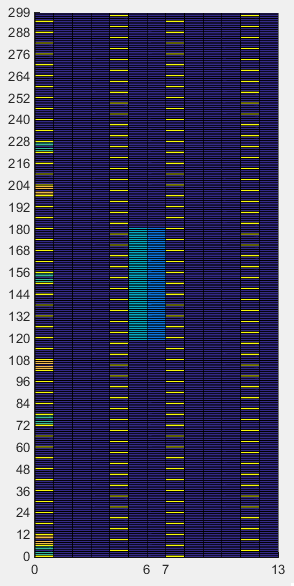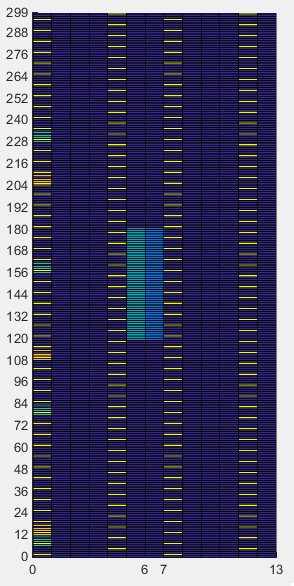Disclaimer ! :

This page is only to show you the overall logics and visualization for various LTE physical layer channels. I haven't investigated much about verifying about the accuracy.

If you think the code is not so efficient, it is 100% my fault. I haven't made any effort for effiecient code. I just tried to create code as simple as possible for the readers. As you know, easy-to-read code is not always efficient for a specific chipset.

If you find any mistake in terms of accuracy, it is also very highly likely be my fault. Not the problem of Matlab tool box itself.

Any comment and corrections if you find any mistake will be welcome and appreciated.